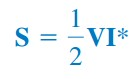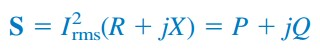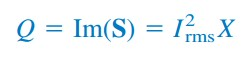omG8HI9LSKFW6lhCj8prTs0Z6lUhdOT9Jhi1Sf4m

# Definition Power Triangle and Complex Power AC Circuits

Considerable effort has been expended over the years to express power relations as simply as possible. Power engineers have coined the term complex power, which they use to find the total effect of parallel loads.

Complex power is important in power analysis because it contains all the information pertaining to the power absorbed by a given load.
Make sure to read what is ac circuit first.

## Complex Power

Consider the ac load in Figure.(1).Figure 1. The voltage and current phasors associated with a load
Given the phasor form V = Vm∠θv and I = Im∠θi of voltage v(t) and current i(t), the complex power S absorbed by the ac load is the product of the voltage and the complex conjugate of the current, or(1)
assuming the passive sign convention (see Figure.1). In terms of the rms values,(2)
where(3)
and(4)
Thus we may write Equation.(2) as(5)
We notice from Equation.(5) that the magnitude of the complex power is the apparent power; hence, the complex power is measured in volt-amperes (VA). Also, we notice that the angle of the complex power is the power factor angle.

The complex power may be expressed in terms of the load impedance Z. The load impedance Z may be written as(6)
Thus, Vrms = ZIrms. Substituting this into Equation.(2) gives(7)
Since Z = R + jX, Equation.(7) becomes(8)
where P and Q are the real and imaginary parts of the complex power; that is,(9)(10)
P is the average or real power and it depends on the load's resistance R. Q depends on the load's reactance X and is called the reactive (or quadrature) power.

Comparing Equation.(5) with (8), we notice that(11)
The real power P is the average power in watts delivered to a load; it is the only useful power. It is the actual power dissipated by the load. The reactive power Q is a measure of the energy exchange between the source and the reactive part of the load.

The unit of Q is the volt-ampere reactive (VAR) to distinguish it from the real power, whose unit is the watt. It represents a lossless interchange between the load and the source. Notice that :
1. Q = 0 for resistive loads (unity pf)
3. Q > 0 for inductive loads (lagging pf)
Thus,
Complex power (in VA) is the product of the rms voltage phasor and the complex conjugate of the rms current phasor. As a complex quantity, its real part is real power P and its imaginary part is reactive power Q.
Introducing complex power enables us to obtain the real and reactive powers directly from voltage and current phasors.(12)
This shows how the complex power contains all the relevant power information in a given load.

It is a standard practice to represent S, P, and Q in the form of a triangle, known as the power triangle, shown in Figure.(2a). This is similar to the impedance triangle showing the relationship between Z, R, and X, illustrated in Figure.(2b).Figure 2. (a) Power triangle, (b) impedance triangle
The power triangle has four items - the apparent/complex power, real power, reactive power, and the power factor angle. Given two of these items, the other two can easily be obtained from the triangle.

As shown in Figure.(3), when S lies in the first quadrant, we have an inductive load and a lagging pf. When S lies in the fourth quadrant, we have a capacitive load and a leading pf. It is also possible for the complex power to lie in the second or third quadrant. This requires that the load impedance have a negative resistance, which is possible with active circuits.Figure 3. Power triangle

## Complex Power Examples

For better understanding let us review examples below :
1. The voltage across a load is v(t) = 60 cos(ωt - 10o) V and the current through the element in the direction of the voltage drop is i(t) =  1.5 cos(ωt + 50o) A. Find : (a) the complex and apparent powers, (b) the real and reactive powers, and (c) the power factor and the load impedance.
Solution :
(a) For the rms values of the voltage and current, we write
The complex power is
The apparent power is

(b) We can express the complex power in rectangular form as
Since S = P + jQ, the real power is
while the reactive power is

(c) The power factor is
It is leading, because the reactive power is negative. The load impedance is
which is a capacitive impedance.

2. A load Z draws 12 kVA at a power factor of 0.856 lagging from a 120-V rms sinusoidal source. Calculate: (a) the average and reactive powers delivered to the load, (b) the peak current, and (c) the load impedance.
Solution:
(a) Given that, pf = cos θ = 0.856, we obtain the power angle as θ = cos-1 0.856 = 31.13o. If the apparent power is S = 12,000 VA, then the average or real power is
while the reactive power is
(b) Since the pf is lagging, the complex power is
From SVrms I*rms, we obtain
Thus Irms = 100∠31.13o and the peak current is
which is an inductive impedance.

Have you understood what is complex power? Don't forget to share and subscribe! Happy learning!
Reference:  Fundamentals of electric circuits by Charles K. Alexander and Matthew N. O. Sadiku

Untuk Bahasa Indonesia baca Apa itu Daya Kompleks Rangkaian Listrik AC.
Related Posts
SHARE